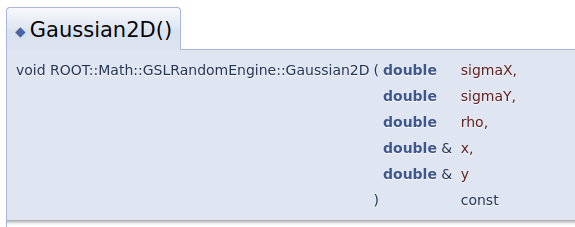# How to get the number of real electrons generated and the electrons collected

like what I typed in the topic, I want to get the totally number of the electrons generated after the proton passing through the gas ionization chamber ne, and in the meanwhile get the number that collected by the collecting plate ne’. Thus I can finally calculate the number of electrons which are recombined with ions or drifting somewhere else.
Now I can have the ne’ data, while to the number of ne I have no idea.

As discussed in the other thread, you can retrieve the coordinates of the end point of each electron trajectory using `GetEndPoint` and then count how many of the electrons ended on the collection electrode.

Hi,
I have calculate the number of electrons generated, the number is 4217,while the endpoint of the electrons is only more than 400.
Why is that?

Besides, how to simulate the incident proton beam that satisfies the Gaussian distribution.

``````heed.SetParticle("p");
heed.SetMomentum(120.e9);
heed.NewTrack(x0, y0, z0, t0, dx0, dy0, dz0);
``````

where x0, y0 are sampled from from a 2D Gaussian distribution,
see e.g. here: ROOT: tutorials/math/multivarGaus.C File Reference

Hi,Thanks a lot for your help.
I am trying to figure it out, while when I try to run the program, there are some warning to notice the .h file not existing or like this

Hi,
the compiler message is quite self-explanatory. Did you try “Gaussian2D” instead of “GaussianND”?

Yep, I have tried, but there is still something wrong

Maybe you need to add `ROOT::MathMore` to the list of libraries in your `CMakeLists.txt`?

``````add_executable(gem gem.C)